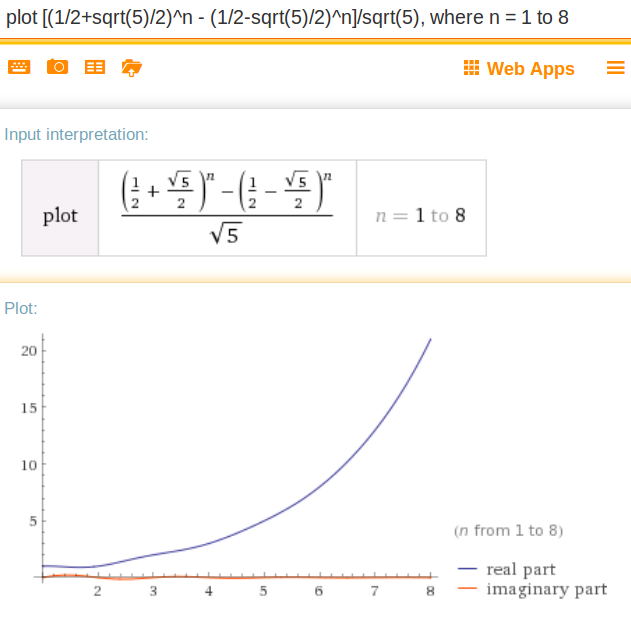# Fibonacci challenge

Hi,

I am working on below challenge
http://codingbat.com/prob/p120015

Psedo code:
1. if first two numbers return same
2. else call same fibonacci method by passing n-1 and n-2 and sum it up

``````public int fibonacci(int n) {

if ((n == 0) || (n == 1)) // base cases
return n;
else
// recursion step
return fibonacci(n - 1) + fibonacci(n - 2);

}
``````

I am passing all tests
Expected      Run
fibonacci(0) → 0      0      OK
fibonacci(1) → 1      1      OK
fibonacci(2) → 1      1      OK
fibonacci(3) → 2      2      OK
fibonacci(4) → 3      3      OK
fibonacci(5) → 5      5      OK
fibonacci(6) → 8      8      OK
fibonacci(7) → 13      13      OK
other tests
OK

How to improve/modify my design, code and any other alternate approaches. please advise
###### Who is Participating?
I wear a lot of hats...

"The solutions and answers provided on Experts Exchange have been extremely helpful to me over the last few years. I wear a lot of hats - Developer, Database Administrator, Help Desk, etc., so I know a lot of things but not a lot about one thing. Experts Exchange gives me answers from people who do know a lot about one thing, in a easy to use platform." -Todd S.

IT Business Systems Analyst / Software DeveloperCommented:
No improvement necessary, that's about as simple as it gets!
Commented:
You could get rid of the extra brackets

Experts Exchange Solution brought to you by

Facing a tech roadblock? Get the help and guidance you need from experienced professionals who care. Ask your question anytime, anywhere, with no hassle.

Senior DeveloperCommented:
This again: These tests and tasks are pretty poor. Fibonacci is definied here over N0. Thus a test covering the input -1 should exist. And you should test the input first.
Commented:
No changes needed - you've nailed this one.

Doug
Commented:
I agree with ste5an. On the other hand, this is for learning the basics of programming, and the totally correct solution (with checking of everything, with unit test, and so on) is not necessary to understand the principle (of Fibonacci, and of the recursive solution).

What is important in this case is that you should not remember this recursive solution as the right approach. The reason is that it is extremely ineficient. The non-recursive solution is not more difficult here, and it is much more efficient.
\Commented:
More advanced ways to compute fibonacci(n) really fast - no recursion, and no loops to get to the nth case.
http://www.maths.surrey.ac.uk/hosted-sites/R.Knott/Fibonacci/fibformproof.html

The way I learned the closed form solution was to take the z-transform of the equation. Here is a link that describes how to do this.
http://mathforum.org/library/drmath/view/51540.html
``````The difference equation for the Fibonacci series is:
u(n+2) = u(n+1) + u(n)   with   u(0) = 0,  u(1) = 1
So:

u(n+2) - u(n+1) - u(n) = 0

Taking the z-transforms:

z^2[u(z) - u(0) - u(1)/z] - z[u(z) - u(0)] - u(z) = 0

u(z)[z^2 - z - 1] - z^2*u(0) - z*u(1) + z*u(0) = 0

Putting u(0) = 0 and u(1) = 1 this becomes:

u(z)[z^2 - z - 1] =  z

z
u(z) =  -------------
z^2 - z - 1

The denominator factorizes to:

[z-1/2 -sqrt(5)/2)][z-1/2 +sqrt(5)/2]

The trick here is to express u(z)/z in partial fractions:

1                     1
u(z)/z =   1/sqrt(5)[ ----------------   - ---------------- ]
z-1/2 - sqrt(5)/2     z-1/2 +sqrt(5)/2

and so:

z                     z
u(z) = 1/sqrt(5)[----------------  - ------------------ ]
z-1/2-sqrt(5)/2)      z-1/2+sqrt(5)/2

Then from table of inverse transforms:

z
-------  =  a^n
z - a

where a is constant. Then:

z                       z
u(z) = 1/sqrt(5) [-------------------- - --------------------]
z - (1/2+sqrt(5)/2)    z - (1/2-sqrt(5)/2)

Now from the table of inverse transforms:
u(n) = 1/sqrt(5)[(1/2+sqrt(5)/2)^n - (1/2-sqrt(5)/2)^n]
``````
\Commented:
Here's a graph of the continuous function (i.e., let n be a continuous real positive number) that I asked wolframalpha to plot.
`````` u(n) = [(1/2+sqrt(5)/2)^n - (1/2-sqrt(5)/2)^n]/sqrt(5)
``````http://www.wolframalpha.com/input/?i=plot+%5B(1%2F2%2Bsqrt(5)%2F2)%5En+-+(1%2F2-sqrt(5)%2F2)%5En%5D%2Fsqrt(5),+where+n+%3D+1+to+8

When n is a positive integer, then u(n) is also a positive integer. Pretty amazing!
In practice, when computing, you probably won't get a positive integer, but a real number that is very close. To get the positive integer, you need to use a round function.
http://www.tutorialspoint.com/java/number_round.htm
\Commented:
As n gets larger, the speed at which you compute fibonacci(n) really rocks over the other approaches, whether recursive or just looping. However, if you want a table of the first N values of fibonacci(n), then just adding the previous two integers and storing it into an array of length N will be faster than using fibonacci(n) for all values of n = 1..N, where n is an integer.
Author Commented:
``````public int fibonacci(int n) {

if ( n == 0 || n == 1) // base cases
return n;
else
// recursion step
return fibonacci(n - 1) + fibonacci(n - 2);

}
``````

got rid of extra brackets. when i have to put and when i can get rid of brackets?
IT Business Systems Analyst / Software DeveloperCommented:
They make absolutely NO difference (in this case) to the execution of the code, if it is easy for you to read with them there, leave them there. That's all that it comes down to, how easy it is to read for YOU.
Commented:
Yes +1 for what mccarl said - I actually think it's a little better with the extra brackets.  You should always prefer clarity and to me the extra with the extra braces is clearer.

Doug
###### It's more than this solution.Get answers and train to solve all your tech problems - anytime, anywhere.Try it for free Edge Out The Competitionfor your dream job with proven skills and certifications.Get started today Stand Outas the employee with proven skills.Start learning today for free Move Your Career Forwardwith certification training in the latest technologies.Start your trial today
Java

From novice to tech pro — start learning today.